Next: Solution of Poisson's equation Up: Particle-in-cell codes Previous: Solution of electron equations

## Evaluation of electron number density

In order to obtain the electron number density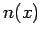from the electron coordinateswe adopt a so-called particle-in-cell (PIC) approach. Let us define a set ofequally spaced spatial grid-points located at coordinates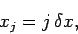(300)

for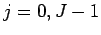, where. Let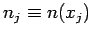. Suppose that the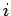th electron lies between the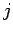th and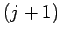th grid-points: i.e.,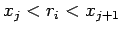. We let(301)

and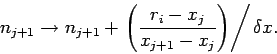(302)

Thus,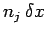increases by 1 if the electron is at theth grid-point,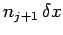increases by 1 if the electron is at theth grid-point, andandboth increase by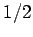if the electron is halfway between the two grid-points, etc. Performing a similar assignment for each electron in turn allows us to build up the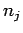from the electron coordinates (assuming that all theare initialized to zero at the start of this process).Next: Solution of Poisson's equation Up: Particle-in-cell codes Previous: Solution of electron equations
Richard Fitzpatrick 2006-03-29